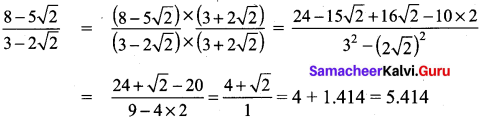# Samacheer Kalvi 9th Maths Solutions Chapter 2 Real Numbers Ex 2.7

## Tamilnadu Samacheer Kalvi 9th Maths Solutions Chapter 2 Real Numbers Ex 2.7

Question 1.
Rationalise the denominator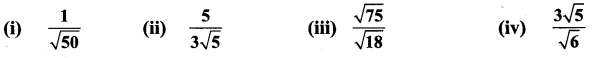Solution: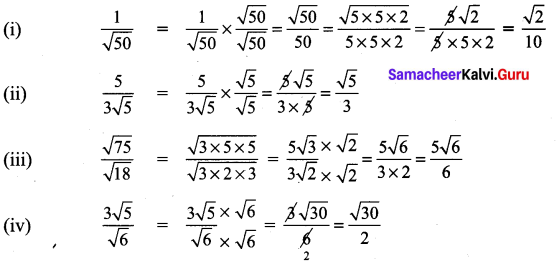Question 2.
Rationalise the denominator and simplify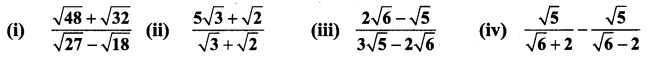Solution: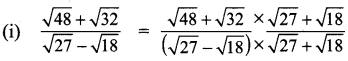Multiply the numerator and denominator by the rationatising factor ($$\sqrt{27}$$ + $$\sqrt{18}$$)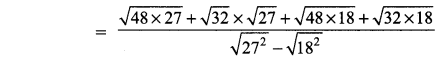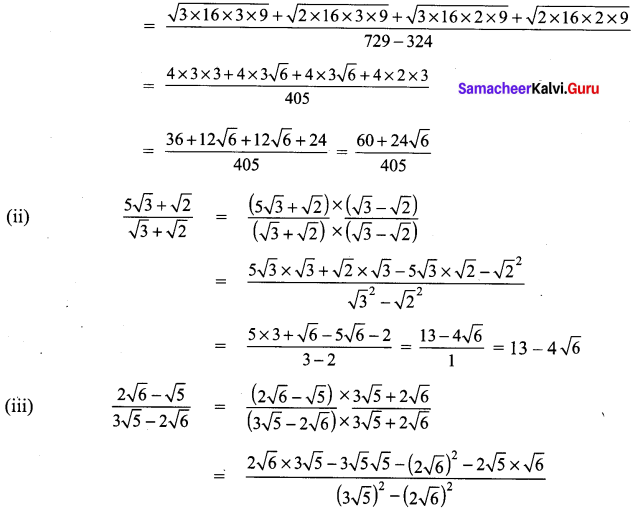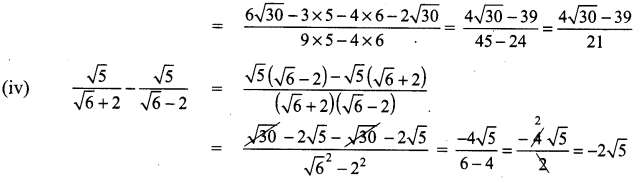Question 3.
Find the value of a and b if $$\frac{\sqrt{7}-2}{\sqrt{7}+2}=a \sqrt{7}+b$$.
Solution: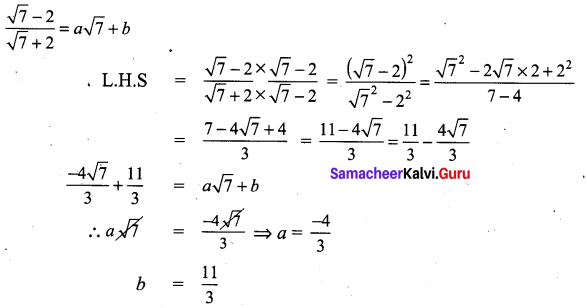Question 4.
If x = $$\sqrt{5}$$ + 2 , then find the value of $$x^{2}+\frac{1}{x^{2}}$$ .
Solution:
If x = $$\sqrt{5}$$ + 2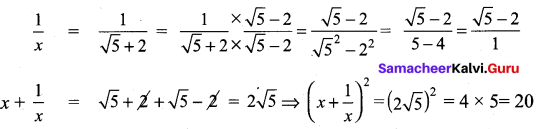Question 5.
Given $$\sqrt{2}$$ = 1.414, find the value of $$\frac{8-5 \sqrt{2}}{3-2 \sqrt{2}}$$ (to 3 places of decimals).
Solution: